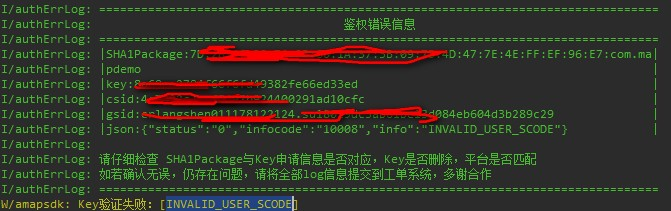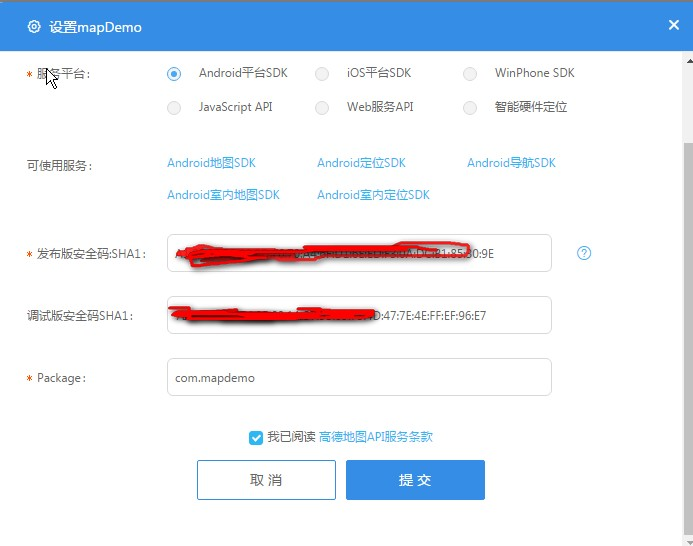• //这里是RSA验证 //这里的字符串是支付宝notfiy 提交上来的 用日志程序把保存到一个文件中 parse_str("total_amount=0.01&buyer_id=2088002088586290&trade_no=2XXXXXXXXXXXXXXXXXXXXXXXXXX",$myArray); //print_r... 终于弄好了。支付宝说明语焉不详啊，我们是做自动售货机软件的，足足花了一天时间啊。。。瞎猜猜对的1.notify 使用的是 支付宝公钥 而不是商户自己的公钥 2.notify 手机支付 使用的是 appid 对应的 支付宝公钥即开放平台的app对应的支付宝公钥 而不是mapi 对应的支付宝公钥1.2018年 支付宝只支持RSA2 2 原来的应用如果申请了。rsa 密钥的话 就是已经启用了rsa ，只能用rsa 。不能同时启用。有折腾一天/* * @author 绍兴远帆软件 http://www.ewshop.net 主营网店系统和自动售货机软件 转载请保留版权 // 文档 https://github.com/lokielse/omnipay-alipay // https://github.com/lokielse/omnipay-alipay/wiki/Aop-WAP-Gateway // https://doc.open.alipay.com/docs/doc.htm?spm=a219a.7629140.0.0.WNXxqz&treeId=193&articleId=105286&docType=1 * notify 调试方法 * 工具：firefox 插件 poster * 网址 http://19.com/alipay/webnotify 参数 tal_amount=0.01&buyer_id=2088002088586290&trade_no=2 一段字符串 为了方便poster 测试 notfify程序中加上 parse_str(file_get_contents("php://input"),$_POST);
*/

//这里是RSA验证

//这里的字符串是支付宝notfiy 提交上来的 用日志程序把保存到一个文件中
parse_str("total_amount=0.01&buyer_id=2088002088586290&trade_no=2XXXXXXXXXXXXXXXXXXXXXXXXXX",$myArray); //print_r($myArray);

$para_temp =$myArray;

$sign =$para_temp['sign'];

//除去待签名参数数组中的空值和签名参数
$para_filter = paraFilter($para_temp);

//var_dump($para_filter); //对待签名参数数组排序$para_sort = argSort($para_filter); //把数组所有元素，按照“参数=参数值”的模式用“&”字符拼接成字符串$prestr = createLinkstring($para_sort); //把数组所有元素，按照“参数=参数值”的模式用“&”字符拼接成字符串$prestr = createLinkstring($para_sort); //var_dump($prestr);

if( rsaVerify($prestr,$sign)){
echo 'ok';
}else{
echo 'fail';
}
/* *
* 支付宝接口公用函数
* 详细：该类是请求、通知返回两个文件所调用的公用函数核心处理文件
* 版本：3.3
* 日期：2012-07-19
* 说明：
* 以下代码只是为了方便商户测试而提供的样例代码，商户可以根据自己网站的需要，按照技术文档编写,并非一定要使用该代码。
* 该代码仅供学习和研究支付宝接口使用，只是提供一个参考。
*/

/**
* 把数组所有元素，按照“参数=参数值”的模式用“&”字符拼接成字符串
* @param $para 需要拼接的数组 * return 拼接完成以后的字符串 */ function createLinkstring($para) {
$arg = ""; while (list ($key, $val) = each ($para)) {
$arg.=$key."=".$val."&"; } //去掉最后一个&字符$arg = substr($arg,0,count($arg)-2);

//如果存在转义字符，那么去掉转义
if(get_magic_quotes_gpc()){$arg = stripslashes($arg);}

return $arg; } /** * 把数组所有元素，按照“参数=参数值”的模式用“&”字符拼接成字符串，并对字符串做urlencode编码 * @param$para 需要拼接的数组
* return 拼接完成以后的字符串
*/
function createLinkstringUrlencode($para) {$arg  = "";
while (list ($key,$val) = each ($para)) {$arg.=$key."=".urlencode($val)."&";
}
//去掉最后一个&字符
$arg = substr($arg,0,count($arg)-2); //如果存在转义字符，那么去掉转义 if(get_magic_quotes_gpc()){$arg = stripslashes($arg);} return$arg;
}
/**
* 除去数组中的空值和签名参数
* @param $para 签名参数组 * return 去掉空值与签名参数后的新签名参数组 */ function paraFilter($para) {
$para_filter = array(); while (list ($key, $val) = each ($para)) {
if($key == "sign" ||$key == "sign_type" || $val == "")continue; else$para_filter[$key] =$para[$key]; } return$para_filter;
}
/**
* 对数组排序
* @param $para 排序前的数组 * return 排序后的数组 */ function argSort($para) {
ksort($para); reset($para);
return $para; } /** * 写日志，方便测试（看网站需求，也可以改成把记录存入数据库） * 注意：服务器需要开通fopen配置 * @param$word 要写入日志里的文本内容 默认值：空值
*/
function logResult($word='') {$fp = fopen("log.txt","a");
flock($fp, LOCK_EX) ; fwrite($fp,"执行日期：".strftime("%Y%m%d%H%M%S",time())."\n".$word."\n"); flock($fp, LOCK_UN);
fclose($fp); } /** * 远程获取数据，POST模式 * 注意： * 1.使用Crul需要修改服务器中php.ini文件的设置，找到php_curl.dll去掉前面的";"就行了 * 2.文件夹中cacert.pem是SSL证书请保证其路径有效，目前默认路径是：getcwd().'\\cacert.pem' * @param$url 指定URL完整路径地址
* @param $cacert_url 指定当前工作目录绝对路径 * @param$para 请求的数据
* @param $input_charset 编码格式。默认值：空值 * return 远程输出的数据 */ function getHttpResponsePOST($url, $cacert_url,$para, $input_charset = '') { if (trim($input_charset) != '') {
$url =$url."_input_charset=".$input_charset; }$curl = curl_init($url); curl_setopt($curl, CURLOPT_SSL_VERIFYPEER, true);//SSL证书认证
curl_setopt($curl, CURLOPT_SSL_VERIFYHOST, 2);//严格认证 curl_setopt($curl, CURLOPT_CAINFO,$cacert_url);//证书地址 curl_setopt($curl, CURLOPT_HEADER, 0 ); // 过滤HTTP头
curl_setopt($curl,CURLOPT_RETURNTRANSFER, 1);// 显示输出结果 curl_setopt($curl,CURLOPT_POST,true); // post传输数据
curl_setopt($curl,CURLOPT_POSTFIELDS,$para);// post传输数据
$responseText = curl_exec($curl);
//var_dump( curl_error($curl) );//如果执行curl过程中出现异常，可打开此开关，以便查看异常内容 curl_close($curl);

return $responseText; } /** * 远程获取数据，GET模式 * 注意： * 1.使用Crul需要修改服务器中php.ini文件的设置，找到php_curl.dll去掉前面的";"就行了 * 2.文件夹中cacert.pem是SSL证书请保证其路径有效，目前默认路径是：getcwd().'\\cacert.pem' * @param$url 指定URL完整路径地址
* @param $cacert_url 指定当前工作目录绝对路径 * return 远程输出的数据 */ function getHttpResponseGET($url,$cacert_url) {$curl = curl_init($url); curl_setopt($curl, CURLOPT_HEADER, 0 ); // 过滤HTTP头
curl_setopt($curl,CURLOPT_RETURNTRANSFER, 1);// 显示输出结果 curl_setopt($curl, CURLOPT_SSL_VERIFYPEER, true);//SSL证书认证
curl_setopt($curl, CURLOPT_SSL_VERIFYHOST, 2);//严格认证 curl_setopt($curl, CURLOPT_CAINFO,$cacert_url);//证书地址$responseText = curl_exec($curl); //var_dump( curl_error($curl) );//如果执行curl过程中出现异常，可打开此开关，以便查看异常内容
curl_close($curl); return$responseText;
}

/**
* 实现多种字符编码方式
* @param $input 需要编码的字符串 * @param$_output_charset 输出的编码格式
* @param $_input_charset 输入的编码格式 * return 编码后的字符串 */ function charsetEncode($input,$_output_charset ,$_input_charset) {
$output = ""; if(!isset($_output_charset) )$_output_charset =$_input_charset;
if($_input_charset ==$_output_charset || $input ==null ) {$output = $input; } elseif (function_exists("mb_convert_encoding")) {$output = mb_convert_encoding($input,$_output_charset,$_input_charset); } elseif(function_exists("iconv")) {$output = iconv($_input_charset,$_output_charset,$input); } else die("sorry, you have no libs support for charset change."); return$output;
}
/**
* 实现多种字符解码方式
* @param $input 需要解码的字符串 * @param$_output_charset 输出的解码格式
* @param $_input_charset 输入的解码格式 * return 解码后的字符串 */ function charsetDecode($input,$_input_charset ,$_output_charset) {
$output = ""; if(!isset($_input_charset) )$_input_charset =$_input_charset ;
if($_input_charset ==$_output_charset || $input ==null ) {$output = $input; } elseif (function_exists("mb_convert_encoding")) {$output = mb_convert_encoding($input,$_output_charset,$_input_charset); } elseif(function_exists("iconv")) {$output = iconv($_input_charset,$_output_charset,$input); } else die("sorry, you have no libs support for charset changes."); return$output;
}

/**
* 验证签名
* @param $prestr 需要签名的字符串 * @param$sign 签名结果
* return 签名结果
*/
function rsaVerify($prestr,$sign) {
$sign = base64_decode($sign);
$public_key= file_get_contents('rsa_public_key.pem');$pkeyid = openssl_get_publickey($public_key); if ($pkeyid) {
$verify = openssl_verify($prestr, $sign,$pkeyid);
openssl_free_key($pkeyid); }else{ echo '公钥格式错误'; } echo$verify;
if($verify == 1){ return true; }else{ return false; } } 第二部分支付宝RSA流程 /* * 服务器异步通知（notify_url）是支付宝服务器主动向商户发送的通知，只有当订单的交易状态改变时才会触发，可以防止因网络等原因引起的丢单问题。对同步通知和异步通知结果的处理步骤大致相同，只是处理完成后的返回值不同。 1. 验证签名 在通知返回参数列表中，除去sign、sign_type两个参数外，凡是通知返回回来的参数皆是要签名的参数，具体的签名步骤与请求时相同。 如果新得到的签名与支付宝返回的签名相同，则签名验证成功。 2. 判断是否是支付宝服务器发来的处理结果 为了防止某些人伪造支付宝发来的处理消息，还要验证消息的合法性，有以下三个方法： 2.1验证消息是否是支付宝发出 paraArray notify_id sign 2.2 活动是否是支付宝服务器发来的青球员的验证结果 notify_id partner https://mapi.alipay.com/gateway.do?partner=合作者身份ID¬ify_id=通知ID的值 2.3 获得远程服务器的ATN结果 strUrl timeout 第五步：需要严格按照如下描述校验通知数据的正确性。 1、商户需要验证该通知数据中的out_trade_no是否为商户系统中创建的订单号， 2、判断total_amount是否确实为该订单的实际金额（即商户订单创建时的金额）， 3、校验通知中的seller_id（或者seller_email) 是否为out_trade_no这笔单据的对应的操作方（ 有的时候，一个商户可能有多个seller_id/seller_email）， 4、验证app_id是否为该商户本身。上述1、2、3、4有任何一个验证不通过，则表明本次通知是异常通知， 务必忽略。在上述验证通过后商户必须根据支付宝不同类型的业务通知，正确的进行不同的业务处理， 并且过滤重复的通知结果数据。在支付宝的业务通知中，只有交易通知状态为TRADE_SUCCESS或TRADE_FINISHED时， 支付宝才会认定为买家付款成功。 * * */ 展开全文• 使用hibernate 连接oracle12c时报名如下 ORA-28040: 没有匹配验证协议 修改文件即可解决:$ORACLE_HOME/network/admin/sqlnet.ora  加入如下:  SQLNET.ALLOWED_LOGON_VERSION=8   ...
使用hibernate 连接oracle12c时报名如下 ORA-28040: 没有匹配的验证协议
修改文件即可解决:
$ORACLE_HOME/network/admin/sqlnet.ora 加入如下: SQLNET.ALLOWED_LOGON_VERSION=8  展开全文• 判断是否是支付宝服务器发来的处理结果 为了防止某些人伪造支付宝发来的处理消息，还要验证消息的合法性，有以下三个方法： 2.1验证消息是否是支付宝发出 paraArray notify_id sign 2.2 活动是否是支付宝服务器发来... 终于弄好了。支付宝说明语焉不详啊，我们是做自动售货机软件的，足足花了一天时间啊。。。瞎猜猜对的1.notify 使用的是 支付宝公钥 而不是商户自己的公钥2.notify 手机支付 使用的是 appid 对应的 支付宝公钥即开放平台的app对应的支付宝公钥而不是mapi 对应的支付宝公钥/** @author 绍兴远帆软件 http://www.ewshop.net 主营网店系统和自动售货机软件转载请保留版权// 文档 https://github.com/lokielse/omnipay-alipay// https://github.com/lokielse/omnipay-alipay/wiki/Aop-WAP-Gateway// https://doc.open.alipay.com/docs/doc.htm?spm=a219a.7629140.0.0.WNXxqz&treeId=193&articleId=105286&docType=1* notify 调试方法* 工具：firefox 插件 poster* 网址 http://19.com/alipay/webnotify参数 tal_amount=0.01&buyer_id=2088002088586290&trade_no=2 一段字符串为了方便poster 测试notfify程序中加上 parse_str(file_get_contents("php://input"),$_POST);*///这里是RSA验证//这里的字符串是支付宝notfiy 提交上来的 用日志程序把保存到一个文件中parse_str("total_amount=0.01&buyer_id=2088002088586290&trade_no=2XXXXXXXXXXXXXXXXXXXXXXXXXX",$myArray);//print_r($myArray);$para_temp =$myArray;$sign =$para_temp['sign'];//除去待签名参数数组中的空值和签名参数$para_filter = paraFilter($para_temp);//var_dump($para_filter);//对待签名参数数组排序$para_sort = argSort($para_filter);//把数组所有元素，按照“参数=参数值”的模式用“&”字符拼接成字符串$prestr = createLinkstring($para_sort);//把数组所有元素，按照“参数=参数值”的模式用“&”字符拼接成字符串$prestr = createLinkstring($para_sort);//var_dump($prestr);if( rsaVerify($prestr,$sign)){echo 'ok';}else{echo 'fail';}/* ** 支付宝接口公用函数* 详细：该类是请求、通知返回两个文件所调用的公用函数核心处理文件* 版本：3.3* 日期：2012-07-19* 说明：* 以下代码只是为了方便商户测试而提供的样例代码，商户可以根据自己网站的需要，按照技术文档编写,并非一定要使用该代码。* 该代码仅供学习和研究支付宝接口使用，只是提供一个参考。*//*** 把数组所有元素，按照“参数=参数值”的模式用“&”字符拼接成字符串* @param $para 需要拼接的数组* return 拼接完成以后的字符串*/function createLinkstring($para) {$arg = "";while (list ($key, $val) = each ($para)) {$arg.=$key."=".$val."&";}//去掉最后一个&字符$arg = substr($arg,0,count($arg)-2);//如果存在转义字符，那么去掉转义if(get_magic_quotes_gpc()){$arg = stripslashes($arg);}return $arg;}/*** 把数组所有元素，按照“参数=参数值”的模式用“&”字符拼接成字符串，并对字符串做urlencode编码* @param$para 需要拼接的数组* return 拼接完成以后的字符串*/function createLinkstringUrlencode($para) {$arg  = "";while (list ($key,$val) = each ($para)) {$arg.=$key."=".urlencode($val)."&";}//去掉最后一个&字符$arg = substr($arg,0,count($arg)-2);//如果存在转义字符，那么去掉转义if(get_magic_quotes_gpc()){$arg = stripslashes($arg);}return$arg;}/*** 除去数组中的空值和签名参数* @param $para 签名参数组* return 去掉空值与签名参数后的新签名参数组*/function paraFilter($para) {$para_filter = array();while (list ($key, $val) = each ($para)) {if($key == "sign" ||$key == "sign_type" || $val == "")continue;else$para_filter[$key] =$para[$key];}return$para_filter;}/*** 对数组排序* @param $para 排序前的数组* return 排序后的数组*/function argSort($para) {ksort($para);reset($para);return $para;}/*** 写日志，方便测试(看网站需求，也可以改成把记录存入数据库)* 注意：服务器需要开通fopen配置* @param$word 要写入日志里的文本内容 默认值：空值*/function logResult($word='') {$fp = fopen("log.txt","a");flock($fp, LOCK_EX) ;fwrite($fp,"执行日期：".strftime("%Y%m%d%H%M%S",time())."\n".$word."\n");flock($fp, LOCK_UN);fclose($fp);}/*** 远程获取数据，POST模式* 注意：* 1.使用Crul需要修改服务器中php.ini文件的设置，找到php_curl.dll去掉前面的";"就行了* 2.文件夹中cacert.pem是SSL证书请保证其路径有效，目前默认路径是：getcwd().'\\cacert.pem'* @param$url 指定URL完整路径地址* @param $cacert_url 指定当前工作目录绝对路径* @param$para 请求的数据* @param $input_charset 编码格式。默认值：空值* return 远程输出的数据*/function getHttpResponsePOST($url, $cacert_url,$para, $input_charset = '') {if (trim($input_charset) != '') {$url =$url."_input_charset=".$input_charset;}$curl = curl_init($url);curl_setopt($curl, CURLOPT_SSL_VERIFYPEER, true);//SSL证书认证curl_setopt($curl, CURLOPT_SSL_VERIFYHOST, 2);//严格认证curl_setopt($curl, CURLOPT_CAINFO,$cacert_url);//证书地址curl_setopt($curl, CURLOPT_HEADER, 0 ); // 过滤HTTP头curl_setopt($curl,CURLOPT_RETURNTRANSFER, 1);// 显示输出结果curl_setopt($curl,CURLOPT_POST,true); // post传输数据curl_setopt($curl,CURLOPT_POSTFIELDS,$para);// post传输数据$responseText = curl_exec($curl);//var_dump( curl_error($curl) );//如果执行curl过程中出现异常，可打开此开关，以便查看异常内容curl_close($curl);return $responseText;}/*** 远程获取数据，GET模式* 注意：* 1.使用Crul需要修改服务器中php.ini文件的设置，找到php_curl.dll去掉前面的";"就行了* 2.文件夹中cacert.pem是SSL证书请保证其路径有效，目前默认路径是：getcwd().'\\cacert.pem'* @param$url 指定URL完整路径地址* @param $cacert_url 指定当前工作目录绝对路径* return 远程输出的数据*/function getHttpResponseGET($url,$cacert_url) {$curl = curl_init($url);curl_setopt($curl, CURLOPT_HEADER, 0 ); // 过滤HTTP头curl_setopt($curl,CURLOPT_RETURNTRANSFER, 1);// 显示输出结果curl_setopt($curl, CURLOPT_SSL_VERIFYPEER, true);//SSL证书认证curl_setopt($curl, CURLOPT_SSL_VERIFYHOST, 2);//严格认证curl_setopt($curl, CURLOPT_CAINFO,$cacert_url);//证书地址$responseText = curl_exec($curl);//var_dump( curl_error($curl) );//如果执行curl过程中出现异常，可打开此开关，以便查看异常内容curl_close($curl);return$responseText;}/*** 实现多种字符编码方式* @param $input 需要编码的字符串* @param$_output_charset 输出的编码格式* @param $_input_charset 输入的编码格式* return 编码后的字符串*/function charsetEncode($input,$_output_charset ,$_input_charset) {$output = "";if(!isset($_output_charset) )$_output_charset =$_input_charset;if($_input_charset ==$_output_charset || $input ==null ) {$output = $input;} elseif (function_exists("mb_convert_encoding")) {$output = mb_convert_encoding($input,$_output_charset,$_input_charset);} elseif(function_exists("iconv")) {$output = iconv($_input_charset,$_output_charset,$input);} else die("sorry, you have no libs support for charset change.");return$output;}/*** 实现多种字符解码方式* @param $input 需要解码的字符串* @param$_output_charset 输出的解码格式* @param $_input_charset 输入的解码格式* return 解码后的字符串*/function charsetDecode($input,$_input_charset ,$_output_charset) {$output = "";if(!isset($_input_charset) )$_input_charset =$_input_charset ;if($_input_charset ==$_output_charset || $input ==null ) {$output = $input;} elseif (function_exists("mb_convert_encoding")) {$output = mb_convert_encoding($input,$_output_charset,$_input_charset);} elseif(function_exists("iconv")) {$output = iconv($_input_charset,$_output_charset,$input);} else die("sorry, you have no libs support for charset changes.");return$output;}/*** 验证签名* @param $prestr 需要签名的字符串* @param$sign 签名结果* return 签名结果*/function rsaVerify($prestr,$sign) {$sign = base64_decode($sign);$public_key= file_get_contents('rsa_public_key.pem');$pkeyid = openssl_get_publickey($public_key);if ($pkeyid) {$verify = openssl_verify($prestr, $sign,$pkeyid);openssl_free_key($pkeyid);}else{echo '公钥格式错误';}echo$verify;if($verify == 1){return true;}else{return false;}}第二部分支付宝RSA流程/**服务器异步通知(notify_url)是支付宝服务器主动向商户发送的通知，只有当订单的交易状态改变时才会触发，可以防止因网络等原因引起的丢单问题。对同步通知和异步通知结果的处理步骤大致相同，只是处理完成后的返回值不同。1. 验证签名在通知返回参数列表中，除去sign、sign_type两个参数外，凡是通知返回回来的参数皆是要签名的参数，具体的签名步骤与请求时相同。如果新得到的签名与支付宝返回的签名相同，则签名验证成功。2. 判断是否是支付宝服务器发来的处理结果为了防止某些人伪造支付宝发来的处理消息，还要验证消息的合法性，有以下三个方法：2.1验证消息是否是支付宝发出paraArraynotify_idsign2.2 活动是否是支付宝服务器发来的青球员的验证结果notify_id partnerhttps://mapi.alipay.com/gateway.do?partner=合作者身份ID¬ify_id=通知ID的值2.3 获得远程服务器的ATN结果strUrltimeout第五步：需要严格按照如下描述校验通知数据的正确性。1、商户需要验证该通知数据中的out_trade_no是否为商户系统中创建的订单号，2、判断total_amount是否确实为该订单的实际金额(即商户订单创建时的金额)，3、校验通知中的seller_id(或者seller_email) 是否为out_trade_no这笔单据的对应的操作方(有的时候，一个商户可能有多个seller_id/seller_email)，4、验证app_id是否为该商户本身。上述1、2、3、4有任何一个验证不通过，则表明本次通知是异常通知，务必忽略。在上述验证通过后商户必须根据支付宝不同类型的业务通知，正确的进行不同的业务处理，并且过滤重复的通知结果数据。在支付宝的业务通知中，只有交易通知状态为TRADE_SUCCESS或TRADE_FINISHED时，支付宝才会认定为买家付款成功。** */ 展开全文• 高德地图继续爬坑 出现上述错误，应该是在打包运行的是debug版本的包，可以高德地图的官网...告诉一个小技巧：直接把log中不匹配的sha1值copy到调试版安全码SHA1中，那下次就直接运行debug版本的包就可以了 高德地图继续爬坑出现上述错误，应该是在打包运行的是debug版本的包，可以高德地图的官网配置。告诉一个小技巧：直接把log中不匹配的sha1值copy到调试版安全码SHA1中，那下次就直接运行debug版本的包就可以了  展开全文sha1 调试 高德地图 • 然后在我们 Model save 时，也会进行 validate 验证验证时，验证码已经重新生成了，所以会匹配不上 // 如果这里用到了验证码，就会出问题$model = new Test(); $model->validate();$model->save(); // 这样是...
• 改了三个小时的Bug呜呜呜终于找出来了 ...2.先检查以下prop的值和model是否匹配：标签里prop参数的命名需要和标签里model数据源对象里的一致才能准确的验证。 3.打开控制台逐一检查是否有一个校验输入符合规则的字符
• 如果 Model 在 save 前，单独做了 validate 验证，则在验证结束后，会重新生成验证码然后在我们 Model save时，也会进行 validate 验证验证时，验证码已经重新生成了，所以会匹配不上// 如果这里用到了验证码，就...
• 在做一个需求时，发现输入正确验证码，但是都提示验证码错误 ...然后在我们 Model save 时，也会进行 validate 验证验证时，验证码已经重新生成了，所以会匹配不上 // 如果这里用到了验证码，就会出问题 ...
• 问题原因：第一次使用Git bash方式拉取代码时，会根据当前的用户和密码生成一串.ssh的密钥，由于每三个月会要求更换一次密码，导致之前生成的密钥无法跟最新的密码匹配，所以出现了可以登录到Git代码仓库，但是在...
• 展开全部刷机包非官方指定的刷机包或者与手机不匹配导致出现该提示，这种情况应该访问小米的e69da5e6ba9062616964757a686964616f31333365633836官方论坛，按照对应的机型选择对应的刷机包，下载到手机或电脑上，然后...
• jetty的端口由80改为8080后，验证ticket异常 org.jasig.cas.client.validation.TicketValidationException 查看cas.log发现url明显不匹配 ERROR [org.jasig.cas.CentralAuthenticationServiceImpl] - Service...
• 6：关于支付签名验证失败 大部分出在二次加密的时候，往上翻，查看第四部分（二次加密），判断加密时参数是否使用错误。 7：![CDATA[sub_mch_id与sub_appid不匹配] 支付相关域名 特约商户APPID配置
• 修改dmp文件里oracle数据库版本号方法，oracle数据库imp导入失败提示：“不是有效的导出文件, 标头验证失败”解决方法。 报错信息如下，该报错主要是因为原来导出时的数据库版本和现有的数据库版本不匹配所致。 解决...
• 1、查看代理主机ip是否正确，fiddler端口与手机设置的端口是否匹配 2、一定记得重新启动fiddler
• RegularExpressionValidator 控件用于验证输入值是否匹配正则表达式指定的模式  属性：  ControlToValidate="要验证的控件名称"  ValidationExpression="验证规则" ... text=“当验证失败时显示的消息。”正则表达式 asp.net asp button server
• 对一个或多个实体的验证失败 https://blog.csdn.net/yanwushu/article/details/48897361 二、导致问题的原因 和朋友交流了，他说: editedPatientInfoTable对象字段过少，与PatientInfo表的字段不匹配。导致...
• RegularExpressionValidator 控件用于验证输入值是否匹配正则表达式指定的模式 属性： ControlToValidate="要验证的控件名称" ValidationExpression="验证规则" Error... text=“当验证失败时显示的消息。” ...
• 宝塔SSL配置教程视频录的一般将就看看吧昨天有人问我SSL怎么配置其实很简单申请下证书就行了第一...crt是证书文件我就随便加一个域名了你们申请的域名和证书一定要匹配找到SSL这个栏目 宝塔在网站设置这里 其他的系...
• 事件消息: 视图状态验证失败。 原因: 提供的视图状态未通过完整性检查。  事件时间: 2011-6-22 16:38:28  事件时间(UTC): 2011-6-22 8:38:28  事件 ID: 63b96ca68f6f4cddb4f4bfb1530920dc  事件序列: 40  ...
• Ubuntu内核自动升级会导致nvidia-smi失败，显卡驱动与内核版本不匹配。解决的两种思路，一是内核版本降级，二是更换与内核版本匹配的驱动。一没有实验成功（可能是配置错误），所以按照二执行，参照网上大神的步骤，...
• 此外，我发现某些电子邮件地址可以使用西里尔文，在所有情况下，标准正则表达式或filter_var()都将失败。这就是我为此制定解决方案的原因：function valid_email($email){if(is_array($email) ...
• 在上一个推送【 我在人民广场吃炸鸡】，上面提过下，最近有空的话，【地图搜租房】小程序会继续做一更新。首当其冲的，大家讨论最多的，估计是搜索功能了。之前在网页端，一早就支持了...搜索匹配的是房源标题，测了...
• 网上找到LDAP用户密码验证的方法，输入正确的密码验证通过，错误的密码便会验证失败，这是符合要求的，但是当密码我给空字符串(“”)的时候竟然也能验证通过，请问原因是什么？我想实...网上找到LDAP用户密码验证的...
• 验证跟踪失败后, 利用关键点匹配的方法对全局关键点进行分类, 根据分类结果对目标关键点进行分析,从而得到重检测结果。实验结果表明, 在运动模糊、变形、目标遮挡、消失等复杂跟踪场景下, 所提算法比现有算法具有...
• java正则验证 String substring = '验证的字符串' String pat = "\\d{4}-\\d{2}-\\d{2}" ; // 指定好正则表达式 ...if(m.matches()){ // 进行验证匹配，使用正则 //通过验证 }else{ //失败 } ...正则表达式 java
• RegularExpressionValidator控件用于验证用户输入值是否匹配正则表达式指定的模式。 　注：如果验证控件为空，则验证不会失败。建议使用RequiredFieldValidator进行非空验证。除非EnabledClientScript属性为false,......